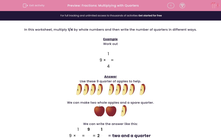# Fractions: Multiplying with Quarters

In this worksheet, students solve simple fraction problems.Key stage:  KS 2

Curriculum topic:   Maths and Numerical Reasoning

Curriculum subtopic:   Fractions

Difficulty level:#### Worksheet Overview

In this worksheet, multiply 1/4 by whole numbers and then write the number of quarters in different ways.

Example

Work out

9  ×
 1 4
=

Use these 9 quarter of apples to help.We can make two whole apples and a spare quarter.We can write the answer like this:

9 ×
 1 4
=
 9 4
= 2
 1 4

= two and a quarter

When we divide 9 by 4, the answer is 2 1/4. The 1 is the remainder and the 4 is the divisor.

Remember also that two quarters make a half.

### What is EdPlace?

We're your National Curriculum aligned online education content provider helping each child succeed in English, maths and science from year 1 to GCSE. With an EdPlace account you’ll be able to track and measure progress, helping each child achieve their best. We build confidence and attainment by personalising each child’s learning at a level that suits them.

Get startedTry an activity or get started for free